A rod of glass ($\upsilon$ = 1.5) and of square cross section is bent into the shape shown in the figure. A parallel beam of light falls on the plane flat surface A as shown in the figure. If d is the width of a side and R is the radius of circular arc then for what maximum value of $\frac{d}{R}$  light entering the glass slab through surface A emerges from the glass through B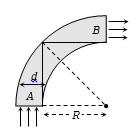(a)   1.5

(b)   0.5

(c)   1.3

(d)   None of these

Concept Questions :-

Total internal reflection
High Yielding Test Series + Question Bank - NEET 2020

Difficulty Level:

A diverging beam of light from a point source S having divergence angle $\alpha$, falls symmetrically on a glass slab as shown. The angles of incidence of the two extreme rays are equal. If the thickness of the glass slab is t and the refractive index n, then the divergence angle of the emergent beam is :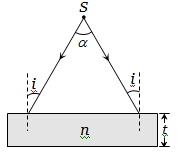(a) Zero                                       (b) $\alpha$

(c) ${\mathrm{sin}}^{-1}\left(1/n\right)$                           (d) $2{\mathrm{sin}}^{-1}\left(1/n\right)$

Concept Questions :-

Refraction at plane surface
High Yielding Test Series + Question Bank - NEET 2020

Difficulty Level:

A concave mirror is placed at the bottom of an empty tank with face upwards and axis vertical. When sunlight falls normally on the mirror, it is focused at distance of 32 cm from the mirror. If the tank is filled with water $\left(\mu =\frac{4}{3}\right)$ upto a height of 20 cm, then the sunlight will now get focussed at

(a) 16 cm above water level

(b) 9 cm above water level

(c) 24 cm below water level

(d) 9 cm below water level

Concept Questions :-

Refraction at plane surface
High Yielding Test Series + Question Bank - NEET 2020

Difficulty Level:

The slab of a material of refractive index 2 shown in figure has curved surface APB of radius of curvature 10 cm and a plane surface CD. On the left of APB is air and on the right of CD is water with refractive indices as given in figure. An object O is placed at a distance of 15 cm from pole P as shown. The distance of the final image of O from P, as viewed from the left is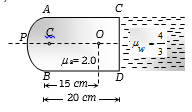(a)   20 cm

(b)   30 cm

(c)   40 cm

(d)   50 cm

Concept Questions :-

Lenses
High Yielding Test Series + Question Bank - NEET 2020

Difficulty Level:

The distance between a convex lens and a plane mirror is 10 cm. The parallel rays incident on the convex lens after reflection from the mirror form image at the optical centre of the lens. Focal length of the lens will be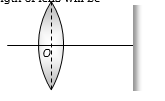(a)   10 cm

(b)   20 cm

(c)   30 cm

(d)   Cannot be determined

Concept Questions :-

Lenses
High Yielding Test Series + Question Bank - NEET 2020

Difficulty Level:

If the focal length of the objective lens and the eye lens is 4 mm and 25 mm respectively in a compound microscope. The length of the tube is 16 cm. Find its magnifying power for relaxed eye position

(a)   32.75                                 (b)  327.5

(c)   0.3275                               (d)  None of the above

Concept Questions :-

Simple and compound microscope
High Yielding Test Series + Question Bank - NEET 2020

Difficulty Level:

An air bubble in a sphere having 4 cm diameter that appears 1 cm from the surface nearest to the eye when looked along diameter. If${}_{a}\mu _{g}$ = 1.5, the distance of bubble from the refracting surface is

(a) 1.2 cm                    (b) 3.2 cm

(c) 2.8 cm                    (d) 1.6 cm

Concept Questions :-

Lenses
High Yielding Test Series + Question Bank - NEET 2020

Difficulty Level:

An observer can see through a pinhole the top end of a thin rod of height h, placed as shown in the figure. The beaker height is 3h and its radius h. When the beaker is filled with a liquid up to a height 2h, he can see the lower end of the rod. Then the refractive index of the liquid is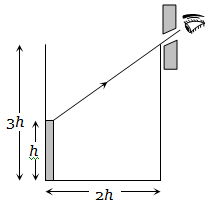(a) $5/2$                                    (b) $\sqrt{\left(5/2\right)}$

(c) $\sqrt{\left(3/2\right)}$                               (d) 3/2

Concept Questions :-

Refraction at plane surface
High Yielding Test Series + Question Bank - NEET 2020

Difficulty Level:

In an experiment of find the focal length of a concave mirror a graph is drawn between the magnitudes of u and v.  The graph looks like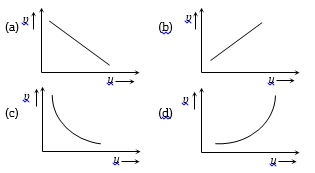Concept Questions :-

Reflection at spherical surface
High Yielding Test Series + Question Bank - NEET 2020

Difficulty Level:

As the position of an object (u) reflected from a concave mirror is varied, the position of the image (v) also varies. By letting the u changes from 0 to  the graph between v versus u will be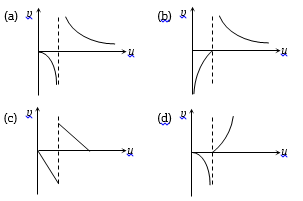Concept Questions :-

Reflection at spherical surface